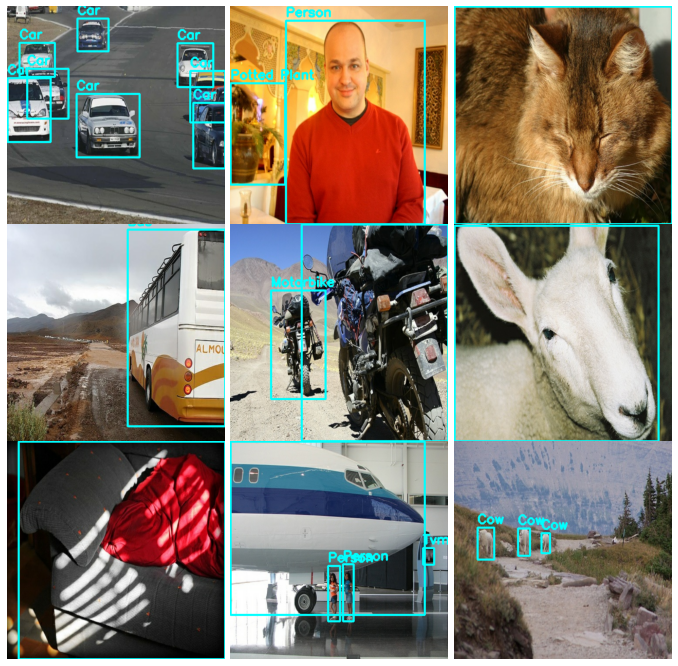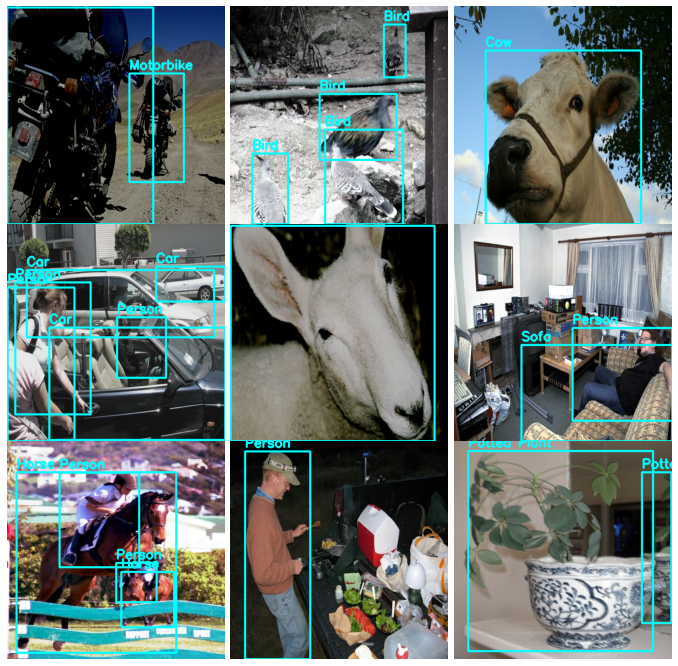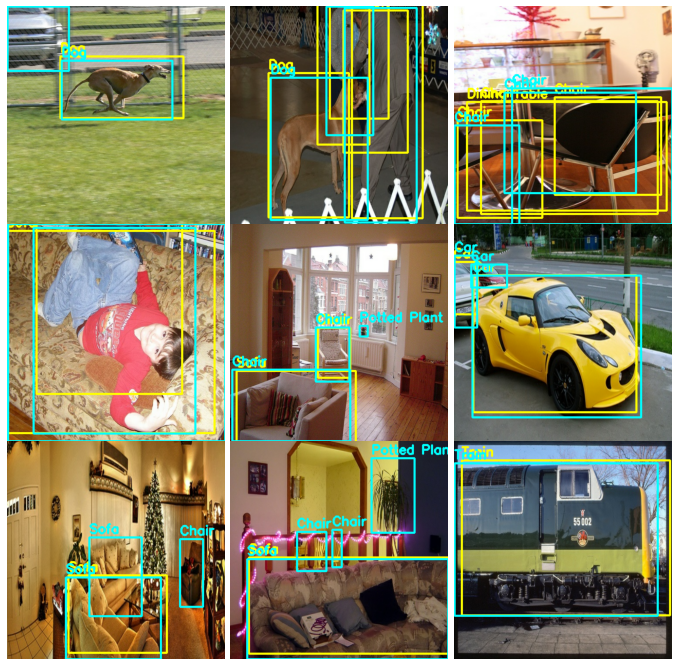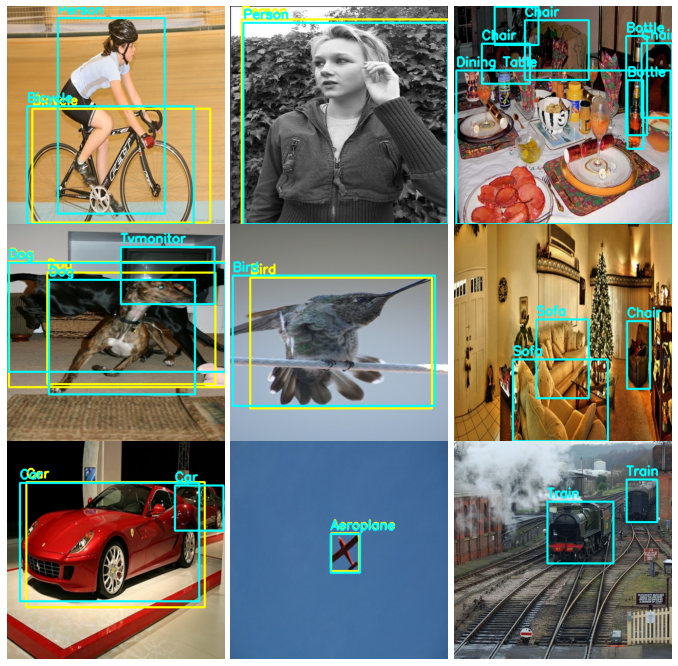» Developer guides / KerasCV / Train an Object Detection Model on Pascal VOC 2007 using KerasCV

# Train an Object Detection Model on Pascal VOC 2007 using KerasCV

Author: lukewood
Date created: 2022/08/22
Description: Use KerasCV to train a RetinaNet on Pascal VOC 2007.View in ColabGitHub source

## Overview

KerasCV offers a complete set of APIs to train your own state-of-the-art, production-grade object detection model. These APIs include object detection specific data augmentation techniques, models, and COCO metrics.

To get started, let's sort out all of our imports and define global configuration parameters.

``````import tensorflow as tf
import tensorflow_datasets as tfds
from tensorflow import keras
from tensorflow.keras import optimizers

import keras_cv
from keras_cv import bounding_box
import os
from luketils import visualization

BATCH_SIZE = 16
EPOCHS = int(os.getenv("EPOCHS", "1"))
CHECKPOINT_PATH = os.getenv("CHECKPOINT_PATH", "checkpoint/")
INFERENCE_CHECKPOINT_PATH = os.getenv("INFERENCE_CHECKPOINT_PATH", CHECKPOINT_PATH)
``````

In this guide, we use the function: `keras_cv.datasets.pascal_voc.load()` to load our data. KerasCV requires a `bounding_box_format` argument in all components that process bounding boxes. To match the KerasCV API style, it is recommended that when writing a custom data loader, you also support a `bounding_box_format` argument. This makes it clear to those invoking your data loader what format the bounding boxes are in.

For example:

``````train_ds, ds_info = keras_cv.datasets.pascal_voc.load(
split='train', bounding_box_format='xywh', batch_size=8
)
``````

Clearly yields bounding boxes in the format `xywh`. You can read more about KerasCV bounding box formats in the API docs.

Our data comesloaded into the format `{"images": images, "bounding_boxes": bounding_boxes}`. This format is supported in all KerasCV preprocessing components.

Let's load some data and verify that our data looks as we expect it to.

``````dataset, dataset_info = keras_cv.datasets.pascal_voc.load(
split="train", bounding_box_format="xywh", batch_size=BATCH_SIZE
)

class_ids = [
"Aeroplane",
"Bicycle",
"Bird",
"Boat",
"Bottle",
"Bus",
"Car",
"Cat",
"Chair",
"Cow",
"Dining Table",
"Dog",
"Horse",
"Motorbike",
"Person",
"Potted Plant",
"Sheep",
"Sofa",
"Train",
"Tvmonitor",
"Total",
]
class_mapping = dict(zip(range(len(class_ids)), class_ids))

def visualize_dataset(dataset, bounding_box_format):
example = next(iter(dataset))
images, boxes = example["images"], example["bounding_boxes"]
visualization.plot_bounding_box_gallery(
images,
value_range=(0, 255),
bounding_box_format=bounding_box_format,
y_true=boxes,
scale=4,
rows=3,
cols=3,
show=True,
thickness=4,
font_scale=1,
class_mapping=class_mapping,
)

visualize_dataset(dataset, bounding_box_format="xywh")
``````Looks like everything is structured as expected. Now we can move on to constructing our data augmentation pipeline.

## Data augmentation

One of the most labor-intensive tasks when constructing object detection pipelines is data augmentation. Image augmentation techniques must be aware of the underlying bounding boxes, and must update them accordingly.

Luckily, KerasCV natively supports bounding box augmentation with its extensive library of data augmentation layers. The code below loads the Pascal VOC dataset, and performs on-the-fly bounding box friendly data augmentation inside of a `tf.data` pipeline.

``````# train_ds is batched as a (images, bounding_boxes) tuple
# bounding_boxes are ragged
train_ds, train_dataset_info = keras_cv.datasets.pascal_voc.load(
bounding_box_format="xywh", split="train", batch_size=BATCH_SIZE
)
val_ds, val_dataset_info = keras_cv.datasets.pascal_voc.load(
bounding_box_format="xywh", split="validation", batch_size=BATCH_SIZE
)

random_flip = keras_cv.layers.RandomFlip(mode="horizontal", bounding_box_format="xywh")
rand_augment = keras_cv.layers.RandAugment(
value_range=(0, 255),
augmentations_per_image=2,
# we disable geometric augmentations for object detection tasks
geometric=False,
)

def augment(inputs):
# In future KerasCV releases, RandAugment will support
# bounding box detection
inputs["images"] = rand_augment(inputs["images"])
inputs = random_flip(inputs)
return inputs

train_ds = train_ds.map(augment, num_parallel_calls=tf.data.AUTOTUNE)
visualize_dataset(train_ds, bounding_box_format="xywh")
``````Great! We now have a bounding box friendly augmentation pipeline.

Next, let's unpackage our inputs from the preprocessing dictionary, and prepare to feed the inputs into our model.

``````def dict_to_tuple(inputs):
return inputs["images"], inputs["bounding_boxes"]

train_ds = train_ds.map(dict_to_tuple, num_parallel_calls=tf.data.AUTOTUNE)
val_ds = val_ds.map(dict_to_tuple, num_parallel_calls=tf.data.AUTOTUNE)

train_ds = train_ds.prefetch(tf.data.AUTOTUNE)
val_ds = val_ds.prefetch(tf.data.AUTOTUNE)
``````

Our data pipeline is now complete. We can now move on to model creation and training.

## Model creation

We'll use the KerasCV API to construct a RetinaNet model. In this tutorial we use a pretrained ResNet50 backbone, initializing the weights to weights produced by training on the imagenet dataset. In order to perform fine-tuning, we freeze the backbone before training. When `include_rescaling=True` is set, inputs to the model are expected to be in the range `[0, 255]`.

``````model = keras_cv.models.RetinaNet(
# number of classes to be used in box classification
classes=20,
# For more info on supported bounding box formats, visit
# https://keras.io/api/keras_cv/bounding_box/
bounding_box_format="xywh",
# KerasCV offers a set of pre-configured backbones
backbone="resnet50",
# Each backbone comes with multiple pre-trained weights
# These weights match the weights available in the `keras_cv.model` class.
backbone_weights="imagenet",
# include_rescaling tells the model whether your input images are in the default
# pixel range (0, 255) or if you have already rescaled your inputs to the range
# (0, 1).  In our case, we feed our model images with inputs in the range (0, 255).
include_rescaling=True,
# Typically, you'll want to set this to False when training a real model.
# evaluate_train_time_metrics=True makes `train_step()` incompatible with TPU,
# and also causes a massive performance hit.  It can, however be useful to produce
# train time metrics when debugging your model training pipeline.
evaluate_train_time_metrics=False,
)
# Fine-tuning a RetinaNet is as simple as setting backbone.trainable = False
model.backbone.trainable = False
``````

That is all it takes to construct a KerasCV RetinaNet. The RetinaNet accepts tuples of dense image Tensors and ragged bounding box Tensors to `fit()` and `train_on_batch()` This matches what we have constructed in our input pipeline above.

## Evaluation with COCO Metrics

KerasCV offers a suite of in-graph COCO metrics that support batch-wise evaluation. More information on these metrics is available in:

Let's construct two COCO metrics, an instance of `keras_cv.metrics.COCOMeanAveragePrecision` with the parameterization to match the standard COCO Mean Average Precision metric, and `keras_cv.metrics.COCORecall` parameterized to match the standard COCO Recall metric.

An important nuance to note is that by default the KerasCV RetinaNet does not evaluate metrics at train time. This is to ensure optimal GPU performance and TPU compatibility. If you want to evaluate train time metrics, you may pass `evaluate_train_time_metrics=True` to the `keras_cv.models.RetinaNet` constructor. Due to this, it is recommended to keep your test set small during training and only evaluate COCO metrics for your full evaluation set as a post-training step.

``````metrics = [
keras_cv.metrics.COCOMeanAveragePrecision(
class_ids=range(20),
bounding_box_format="xywh",
name="Mean Average Precision",
),
keras_cv.metrics.COCORecall(
class_ids=range(20),
bounding_box_format="xywh",
max_detections=100,
name="Recall",
),
]
``````

## Training our model

All that is left to do is train our model. KerasCV object detection models follow the standard Keras workflow, leveraging `compile()` and `fit()`.

Let's compile our model:

``````optimizer = tf.optimizers.SGD(global_clipnorm=10.0)
model.compile(
classification_loss=keras_cv.losses.FocalLoss(from_logits=True, reduction="none"),
box_loss=keras_cv.losses.SmoothL1Loss(l1_cutoff=1.0, reduction="none"),
optimizer=optimizer,
metrics=[
keras_cv.metrics.COCOMeanAveragePrecision(
class_ids=range(20),
bounding_box_format="xywh",
name="Mean Average Precision",
),
keras_cv.metrics.COCORecall(
class_ids=range(20),
bounding_box_format="xywh",
max_detections=100,
name="Recall",
),
],
)
``````

Next, we can construct some callbacks:

``````callbacks = [
keras.callbacks.TensorBoard(log_dir="logs"),
keras.callbacks.ReduceLROnPlateau(patience=5),
# Uncomment to train your own RetinaNet
keras.callbacks.ModelCheckpoint(CHECKPOINT_PATH, save_weights_only=True),
]
``````

And run `model.fit()`!

``````model.fit(
train_ds,
validation_data=val_ds.take(20),
epochs=EPOCHS,
callbacks=callbacks,
)
model.save_weights(CHECKPOINT_PATH)
``````
``````157/157 [==============================] - 180s 1s/step - loss: 26.5893 - classification_loss: 13.6207 - box_loss: 12.9686 - val_Mean Average Precision: 0.0029 - val_Recall: 0.0079 - val_loss: 22.9552 - val_classification_loss: 11.2301 - val_box_loss: 11.7251 - lr: 0.0100
``````

Next, we can evaluate the metrics by re-compiling the model, and running `model.evaluate()`:

``````model.load_weights(INFERENCE_CHECKPOINT_PATH)
metrics = model.evaluate(val_ds.take(100), return_dict=True)
print(metrics)
``````
``````100/100 [==============================] - 391s 4s/step - Mean Average Precision: 0.0912 - Recall: 0.2442 - loss: 11.2131 - classification_loss: 4.8064 - box_loss: 6.4066
{'Mean Average Precision': 0.09121730178594589, 'Recall': 0.24424493312835693, 'loss': 11.213068962097168, 'classification_loss': 4.8064470291137695, 'box_loss': 6.406621932983398}
``````

## Inference

KerasCV makes object detection inference simple. `model.predict(images)` returns a RaggedTensor of bounding boxes. By default, `RetinaNet.predict()` will perform a non max suppression operation for you.

``````def visualize_detections(model, bounding_box_format):
train_ds, val_dataset_info = keras_cv.datasets.pascal_voc.load(
bounding_box_format=bounding_box_format, split="train", batch_size=BATCH_SIZE
)
train_ds = train_ds.map(dict_to_tuple, num_parallel_calls=tf.data.AUTOTUNE)
images, y_true = next(iter(train_ds.take(1)))
y_pred = model.predict(images)
visualization.plot_bounding_box_gallery(
images,
value_range=(0, 255),
bounding_box_format=bounding_box_format,
y_true=y_true,
y_pred=y_pred,
scale=4,
rows=3,
cols=3,
show=True,
thickness=4,
font_scale=1,
class_mapping=class_mapping,
)

visualize_detections(model, bounding_box_format="xywh")
``````
``````1/1 [==============================] - 2s 2s/step
``````To get good results, you should train for at least 100 epochs. You also need to tune the prediction decoder layer. This can be done by passing a custom prediction decoder to the RetinaNet constructor as follows:

``````prediction_decoder = keras_cv.layers.NmsPredictionDecoder(
bounding_box_format="xywh",
anchor_generator=keras_cv.models.RetinaNet.default_anchor_generator(
bounding_box_format="xywh"
),
suppression_layer=keras_cv.layers.NonMaxSuppression(
iou_threshold=0.75,
bounding_box_format="xywh",
classes=20,
confidence_threshold=0.85,
),
)
model.prediction_decoder = prediction_decoder
visualize_detections(model, bounding_box_format="xywh")
``````
``````1/1 [==============================] - 3s 3s/step
``````## Results and conclusions

KerasCV makes it easy to construct state-of-the-art object detection pipelines. All of the KerasCV object detection components can be used independently, but also have deep integration with each other. With KerasCV, bounding box augmentation, train-time COCO metrics evaluation, and more, are all made simple and consistent.

Some follow up exercises for the reader:

• add additional augmentation techniques to improve model performance
• grid search `confidence_threshold` and `iou_threshold` on `NmsPredictionDecoder` to achieve an optimal Mean Average Precision
• tune the hyperparameters and data augmentation used to produce high quality results
• train an object detection model on another dataset